blog

# Bull Call Spread Option Payoff Graph

#### Options Trading 101 - The Ultimate Beginners Guide To Options

As Seen On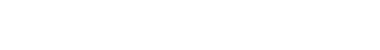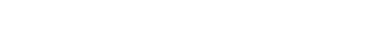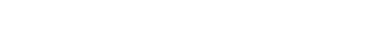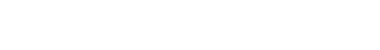by Gavin in Blog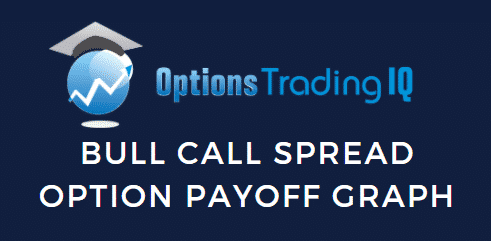Today we’re going to look at the bull call spread option payoff graph. This strategy is also sometimes call a bull debit spread or call debit spread.

This is designed for beginners so they can develop a basic understanding how to read the payoff graphs.

We’ll look at:

## Bull Call Spread Option Basics

A bull call spread is one of the easiest option trades and a great place for beginners to start their learning journey.

A bull call spread option is 2 contracts between a buyer and seller. It is an options strategy that is used when the investor expects a moderate rise in the price of the underlying asset. The strategy uses two call options to form a range consisting of a high strike price and a low strike price

## Bull Call Spread Option Payoff Graph

Understanding payoff graphs (or diagrams as they are sometimes referred) is absolutely essential for option traders.

A payoff graph will show the option position’s total profit or loss (Y-axis) depending on the underlying price (x-axis).

Here is an example: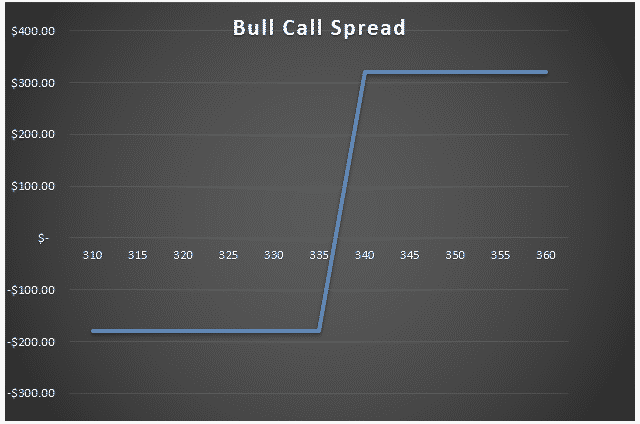What we are looking at here is the payoff graph for a bull call spread option strategy.

In this example the trader has bought a \$335 strike call and sold a \$340 strike call for a net \$1.80 per contract (or \$180 for a standard option contract representing 100 shares).

The premium paid of \$180 is the most the trade can lose. That occurs at an underlying stock price of \$335 and below.

Above the strike price of \$335, the line slopes upward as the stock price rises.

Above \$340 is where the max profit is at expiration.

The lines crosses into profit at a certain point when the stock price rise above the breakeven point.

The breakeven point is simply the long strike price plus the premium paid. In this case it would be: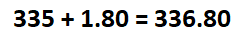If the premium paid had been slightly higher at \$3, then the breakeven price would be \$338

## Different Scenarios At Expiration

Four different scenarios can occur at expiration for a bull call spread trade.

### 1. Underlying stock price is above the short strike price

This is the ideal scenario that the trader was hoping for. If the underlying stock price is above the short strike price at expiration, the entire bull call spread will be in-the-money, the long call will be exercised at \$335 and short call assigned at \$340.

The profit for the trade will be equal to the width of the strikes minus premium paid.

In our example, this would be \$5 – \$1.80 = \$3.20 per contract or \$320 in total.

The trader can either let the options be exercised and assigned automatically by the broker or sell them back to the market for a profit.

### 2. Underlying stock price is below the short strike price but above the breakeven price

If the stock is below the short strike price at expiration, the trader may think the trade has a loss, but unless it gets below the breakeven price, it will not end in a loss.

For example, let’s say the stock ends at \$338.

The \$335 strike long call option has \$3 of profit and it will be exercised. The \$340 strike short call option expired worthless

The \$3 of profit is more than the \$1.80 paid, so in this scenario, the trader has profit \$1.20 per contract.

### 3. Underlying stock price is below the breakeven price but above the long strike price

If the stock is below the breakeven price at expiration, the trader may think the position has a max loss, but unless it gets below the long strike price, it will not end in a max loss.

For example, let’s say the stock ends at \$336.

The \$335 strike long call option has \$1 of profit and it will be exercised. The \$340 strike short call option expired worthless

But, the \$1 of profit minus \$1.80 premium paid is less than the max loss of \$\$1.80 paid, so in this scenario, the trader has loss of only \$0.80 per contract compared to max loss of \$1.80.

### 4. Underlying stock price is lower than the long call price

If the underlying stock price is below the long strike price at expiration, the entire bull call spread option will expire worthless for both strikes. The loss for the trade will be equal to the premium paid.

In our example, this would be \$1.80 per contract or \$180 in total.

The good thing about a bull call spread is that the loss is limited to the premium paid, so it doesn’t matter how low the stock goes, the most the trader can lose is the \$180 in this example.

## Payoff Graph At Interim Dates

So far, we’ve only considered the payoff graph at expiration. The payoff graph at interim dates will look very different and will be impacted by factors such as time decay and implied volatility.

Calculating the profit and loss at expiration is quite simply, using excel or even in your head if you are half decent at maths.

Calculating profits and losses at interim dates is much more complex and requires more advanced software than just Excel.

Any decent options broker will have the ability to show option profits and losses at interim dates.

The below bull call spread option payoff is from Interactive Brokers.

The bull call spread option was an AAPL 115/120 strike call bought at \$1.50 per contract or \$150 in total. The breakeven price at expiration is \$116.50 (long strike price plus the premium paid).

The blue line shows the expiration payoff that you are now familiar with and the purple line shows what is known as a “T+0” line.

T+0 simply means, what does the trade look like as of today?

Notice that the breakeven price as of today is the current AAPL price. This makes sense because we just bought the bull call spread so wouldn’t expect to have made or lost any money yet.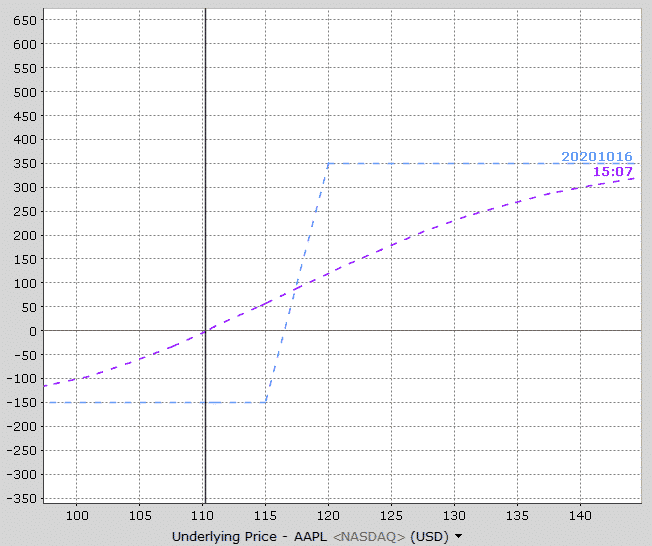Notice also that profits occur much before the expiration breakeven price and above 120, the profits are not the maximum profit.

As time goes by, the interim line slowly moves towards the expiration line.

Here’s how the payoff diagram is estimated to look at one week before expiration.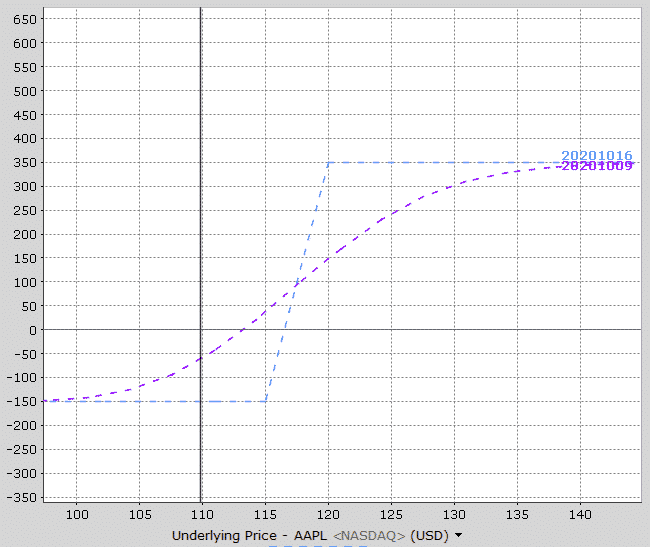See how the purple line is now much closer to the expiration payoff line?

Most brokers will be able to provide this sort of graphical information for you.

I also like to use Option Net Explorer because they can show multiple interim date lines.

Looking at the payoff graph below, notice that there are three interim lines – T+0, T+9 and T+18. This gives the trader a good appreciation of how the trade will progress over time after 9 days and 18 days.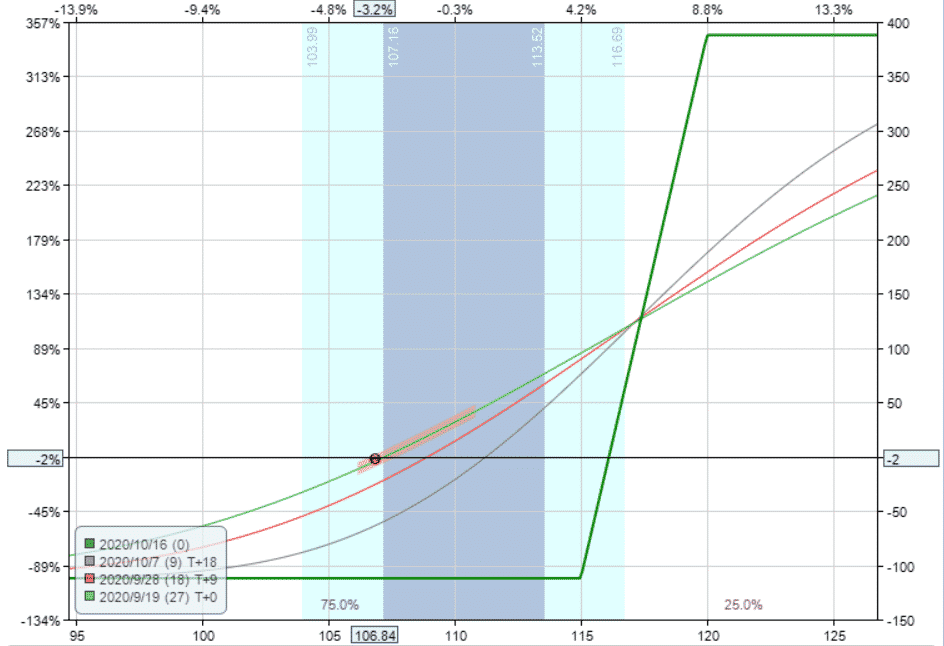## How Changes In Volatility Impact The Payoff Graph

Changes in implied volatility will not have any impact on the payoff graph at expiration, but it will impact the interim dates.

A bull call spread strategy has positive vega, meaning that it will benefit from an increase in implied volatility after placing the trade.

This AAPL trade example has vega of 2 which means that for every 1% rise in implied volatility, the trade will make \$2 with all other factors being equal.

The opposite is true if implied volatility drops.

Let’s take a look at how a 20% rise in volatility impacts the interim lines.

Notice that the expiration line hasn’t changed at all, but the interim lines have changed. The impact of volatility changes depends on where the stock price is.

Below the mid-point of the spread a rise in volatility helps the trade, but above the mid-point of the spread, volatility hurts the trade.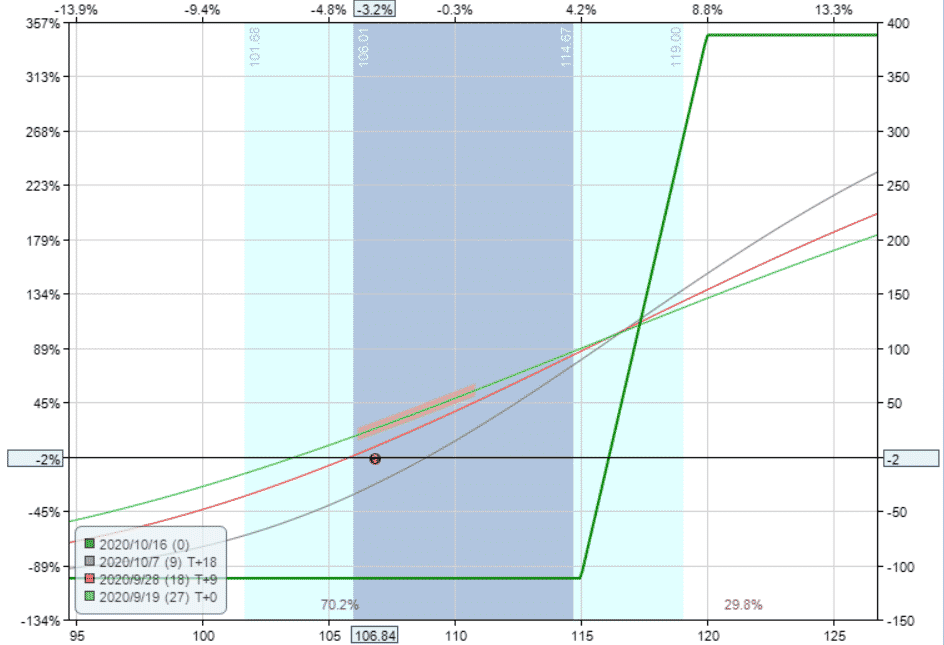The opposite is true if we assume a 20% drop in volatility. Below the mid-point of the spread it hurts, above the mid-point it helps the trade.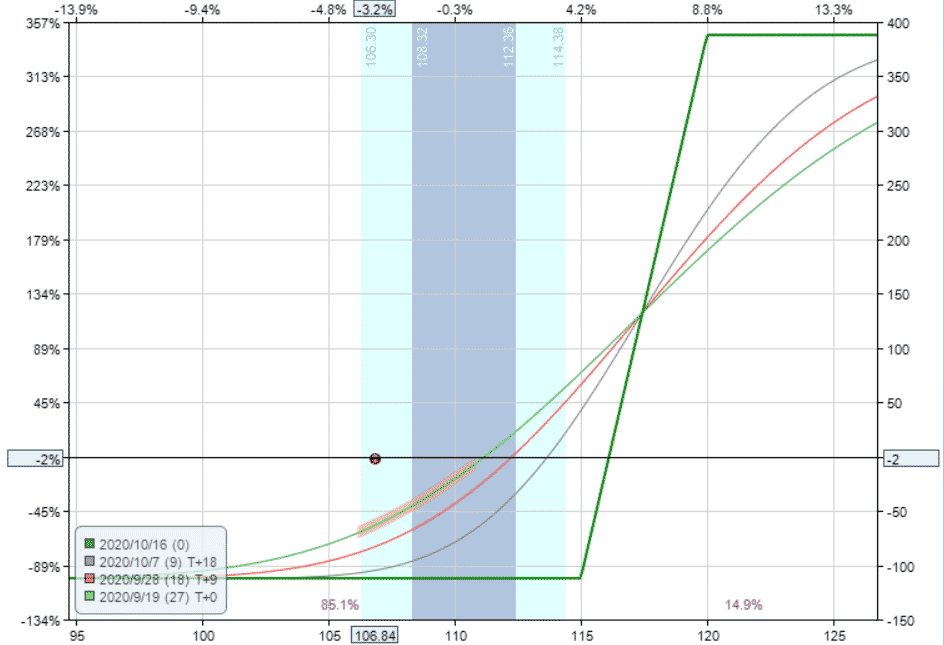With a bull call spread strategy, delta is the main driver of the trade, but we can clearly see that big changes in implied volatility will also affect the trade.

## Bull Call Spread Option Payoff Summary

You should now be intimately familiar with the bull call spread option payoff graph. Let’s summarize some key points.

• Bull call spread options are a bulish strategy with limited risk and limited upside
• The maximum loss is equal to the premium paid
• The breakeven price is equal to the long strike price plus the premium paid
• The position will lose value as expiration approaches due to time decay. But, if the stock is above the mid-point of the spread, time decay actually helps the trade.
• As a positive vega trade, increases in implied volatility will help the trade, but only at interim dates and only when the stock is below the mid-point of the spread

If you’re still having trouble or want to learn more about these concepts, you can check out this 12,000 word beginners guide to options.